Mateusz

# Probability distribution sampling in C# using StatDist and Troschuetz.Random

## Introduction

Let’s talk about sampling probability density functions.

For example, imagine that you’re generating a combat encounter.0 You need to spawn several enemies, but not a fixed count - let’s assume a range of `[1 .. 10]` opponents.

At this point we could roll a d10 and call it a day, but that wouldn’t feel very creative. What if we’d prefer to usually choose a small number of opponents, and only occasionally challenge the player with a larger fight?

Basically, we want to sample a probability distribution that looks like this: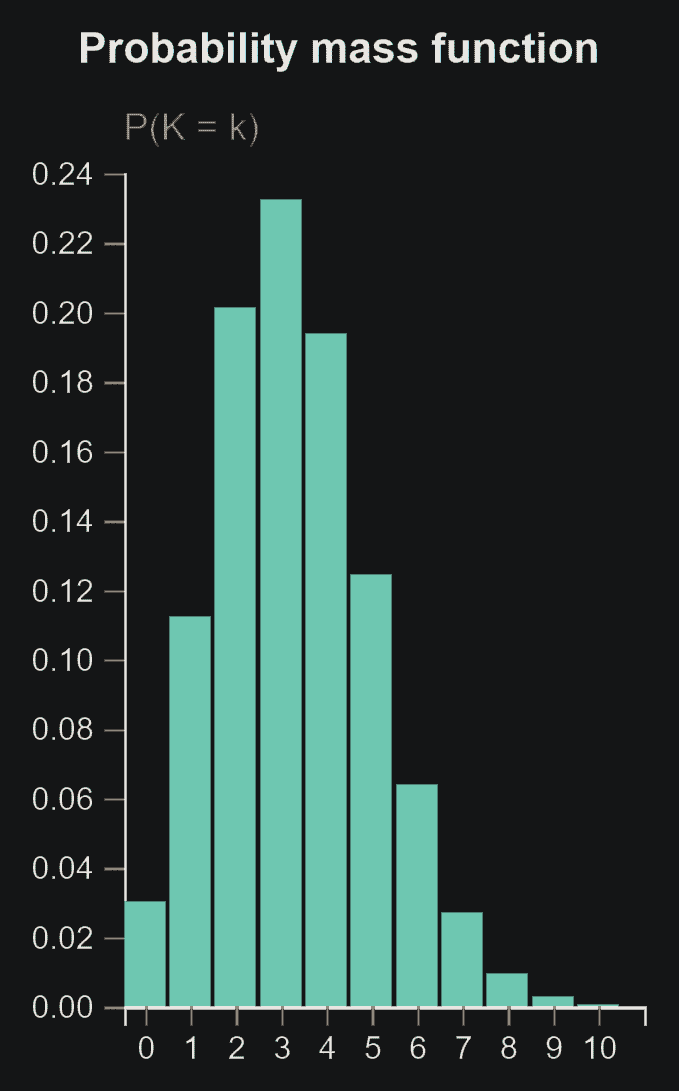Binomial distribution with `n = 30, p = 0.11` - usually yields a number around 3, but sometimes even up to 10.

Different scenario. You’re spawning some loot and need to decide whether to include a rare item. Again, if your game is simple a dice roll might be sufficient. But let’s assume that you wish the player to have a `Luck` stat that affects the drop chance nonlinearly. Consider these constraints:

• At low levels, you want the player to immediately feel the benefits of increasing their `Luck`
• Obviously, the drop probability cannot exceed 100%
• But at the same time it would be nice if stacking huge amounts of `Luck` still benefited the player somewhat, even if by a small amount

What we need is a random event that depends on a difficulty level with diminishing returns. Basically, we’re looking for a distribution with a cumulative distribution that looks something like this: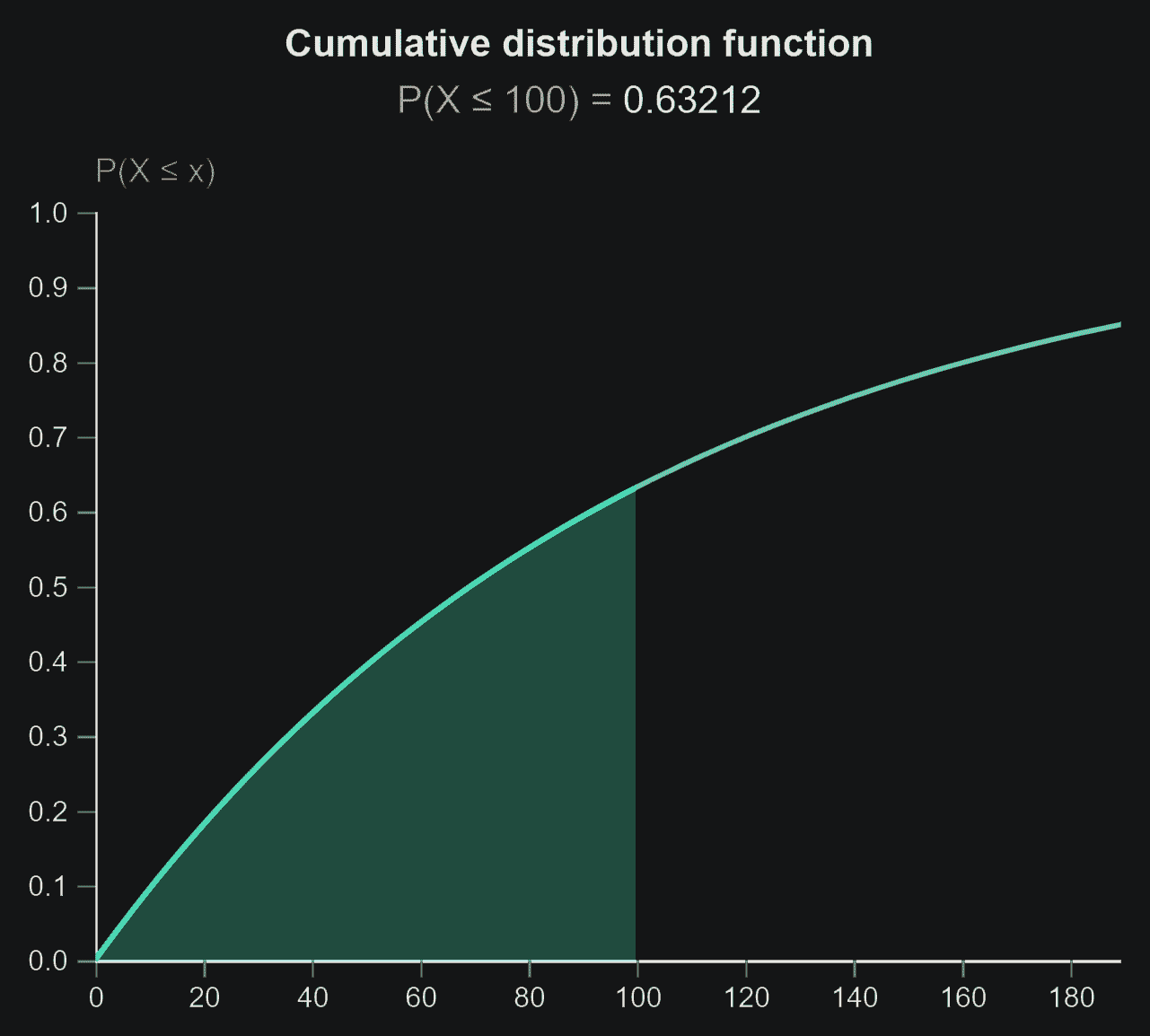Cumulative distribution function for the Erlang distribution with `k = 1, λ = 0.01`.
In our example, 𝑥 represents our character’s `Luck`.
P(𝒳 < 𝑥) - the green area - represents the probability that `Luck` is sufficient to spawn the item.

These are just two examples, but what you probably want is some different probability distribution, with a set of parameters that is specific to your game or program.

If you’re a visual thinker, you might have a rough idea of what shape your imaginary reward value distribution is, but no idea how to represent it in code. How do we solve this? Here’s one workflow I like…

## Finding your dream distribution w/ StatDist

StatDist is a cool little website that lets you plot dozens of common statistical distributions.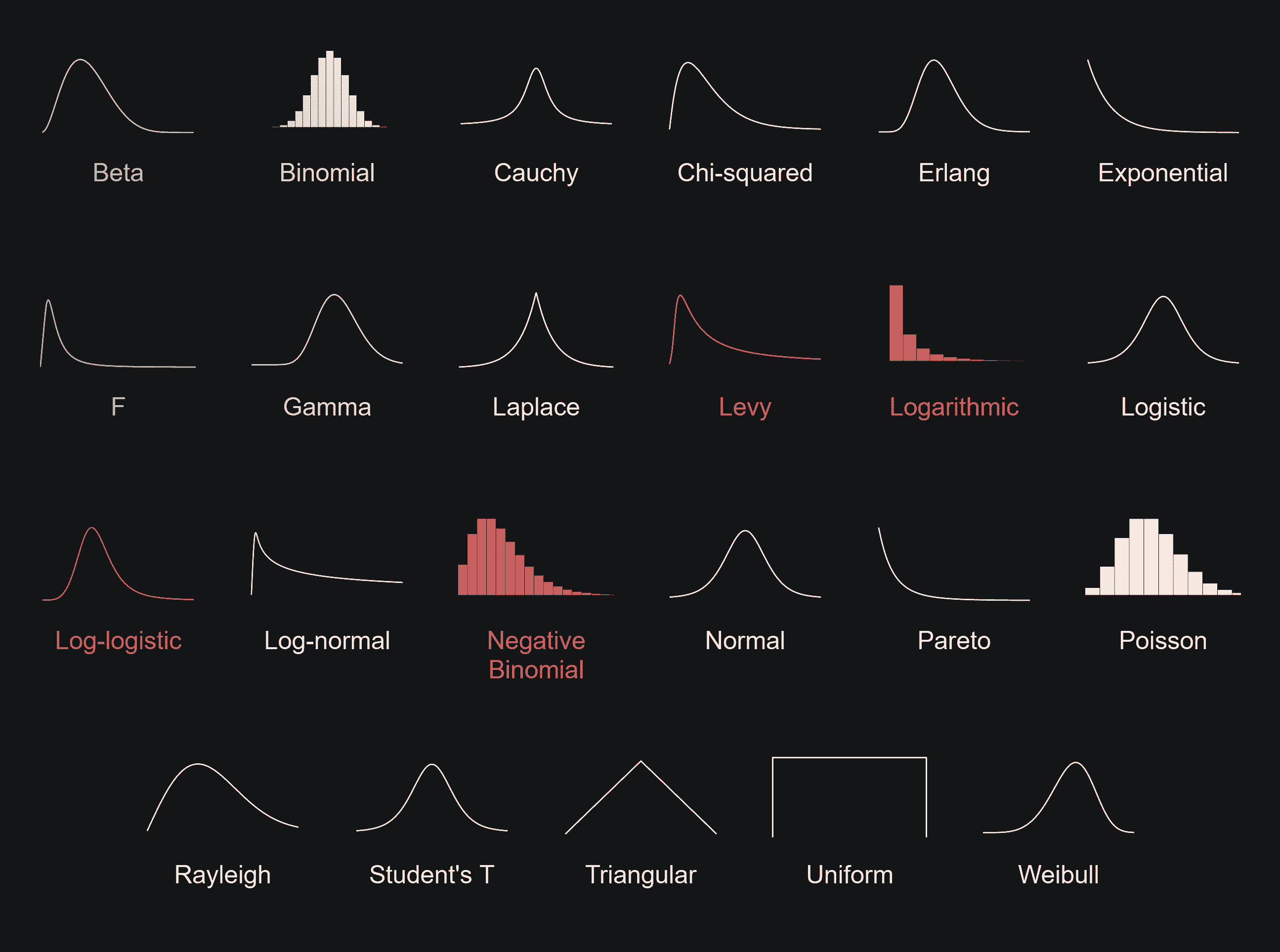Distributions shown in red are not supported by Troschuetz.Random

Simply choose a distribution, input the parameters and you’ll get an overview of what the distribution is like. Thankfully, the scary equations are hidden by default, but there’s a Details button for the brave.

The graphs and the 𝑥 parameter (which lets us preview the value of the function at 𝑥) can be used to build intuition for how the distribution behaves by simply playing with it.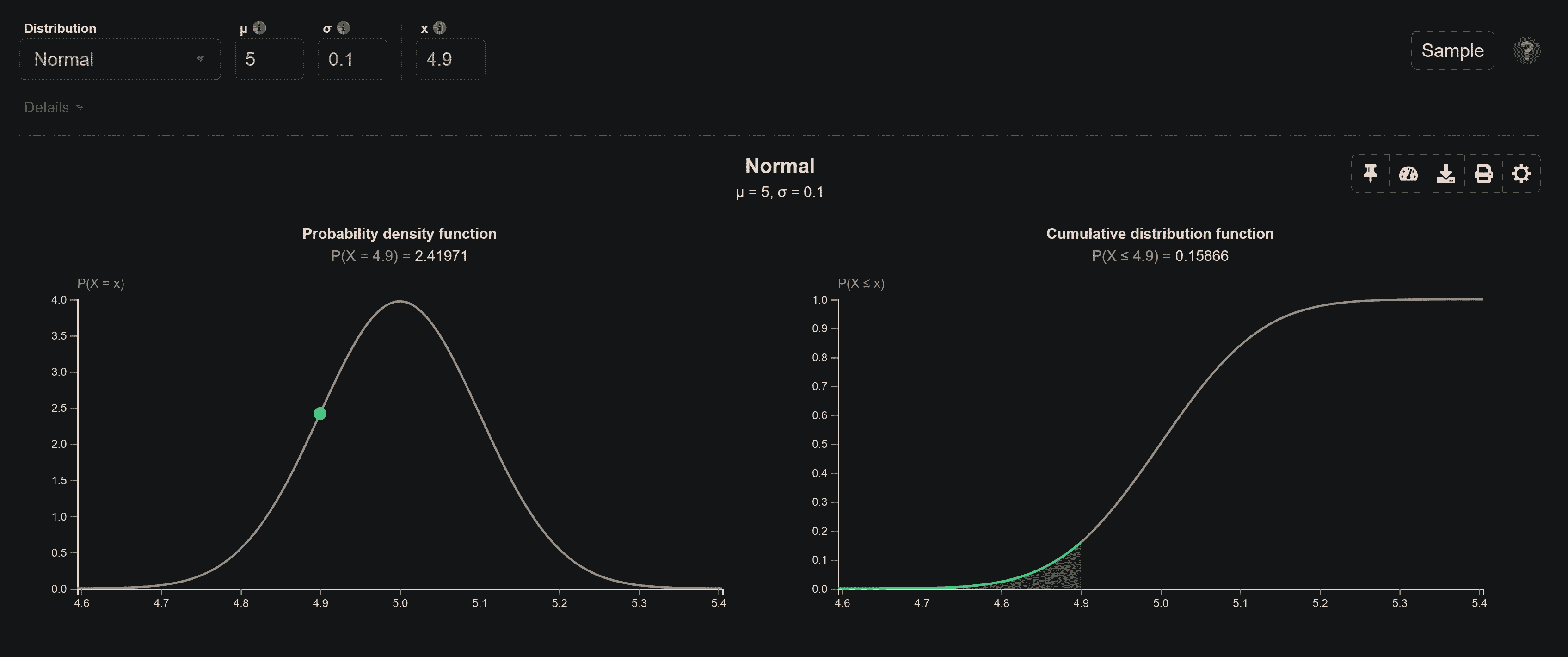## Sampling distributions w/ Troschuetz.Random

Troschuetz.Random is a library which implements various random number generators and distributions. In most cases, you can simply copy the parameters chosen using StatDist into the function call. Super convenient.

``````0
1
2
3
4
5
6
var random = new Troschuetz.Random.TRandom();

// get a random value based on some chosen distribution
double sample = random.Binomial(alpha: 30, beta: 0.1);

// clamp the value to our indended value range (recommended)
sample = Math.Clamp(sample, min: 0, max: 10);
``````

This yields us a random 𝑥 based on the distribution we previously nailed down. No longer are we bound to the uniformly-distributed random numbers generated by `System.Random` - now we can sample any random distribution we like!

## Generating random events w/ Troschuetz.Random

Sometimes we want to decide whether a random event has occured based on a parameter that represents difficulty.1

If you ever used the `System.Random` class like this: `random.Next() < 0.9 // 90% probability`, then this is basically the same thing, except with `TRandom` we can use a more interesting, non-uniform distribution of random values. This allows us to have a non-linear relationship between the difficulty and the probability of the random event.2

Basically, you just do this:

``````0
1
2
3
4
5
6
7
8
9
10
11
var random = new Troschuetz.Random.TRandom();

// the "luck" value that we want our random value to undershoot
// (if you prefer to think in terms of a "difficulty" value instead, simply invert the final result)
double luck = 100;

// get a random value based on some chosen distribution
double sample = random.Erlang(alpha: 1, lambda: 0.01);

// test whether the random value is less than the player's "luck"
// (or higher than the "difficulty" value)
bool randomEventOccurred = sample < luck;
``````

## Distributions supported by both Troschuetz.Random and StatDist

Here’s the functions you can use. Also check out this article by the package author and the API docs.

### `double Beta(double alpha, double beta);`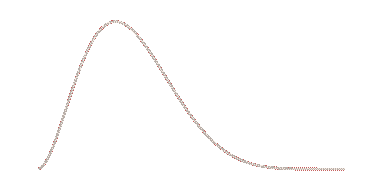0 < α < ∞
0 < β < ∞
0 ≤ X ≤ 1
View on StatDist
View on Wikipedia

### `int Binomial(double alpha /* trials */, int beta /* probability */);`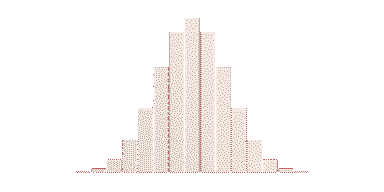0 ≤ α ≤ 1
β ∈ { 0, 1, … }
X ∈ { 0, 1, …, β }
View on StatDist
View on Wikipedia

### `double Cauchy(double alpha /* location */, double gamma /* scale */);`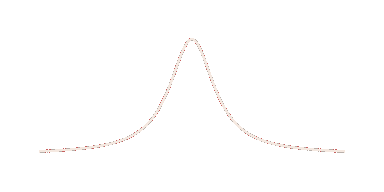-∞ < α < ∞
0 < γ < ∞
-∞ < X < ∞
View on StatDist
View on Wikipedia

### `double ChiSquare(int alpha /* degrees of freedom */);`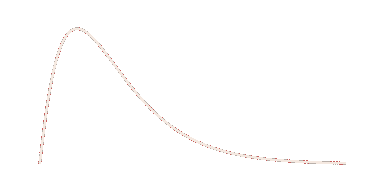α ∈ { 1, 2, … }
0 ≤ X < ∞
View on StatDist
View on Wikipedia

### `double ContinuousUniform(double alpha /* min */, double beta /* max */);`α ≤ β < ∞
-∞ < α ≤ β
α ≤ X < β
View on StatDist
View on Wikipedia

### `int DiscreteUniform(int alpha /* min */, int beta /* min */);`α ∈ { …, β-1, β}
β ∈ { α, α+1, … }
X ∈ { α, α+1, …, β-1, β }
Not on StatDist, but see the continuous version above
View on Wikipedia

### `double Erlang(int alpha /* shape */, double lambda /* rate */);`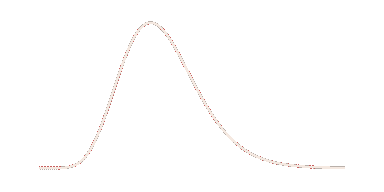0 < α < ∞
λ ∈ { 1, 2, … }
0 ≤ X < ∞
View on StatDist
View on Wikipedia

### `double Exponential(double lambda /* rate */);`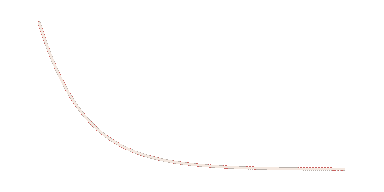0 < λ < ∞
0 ≤ X < ∞
View on StatDist
View on Wikipedia

### `double FisherSnedecor(int alpha, int beta);`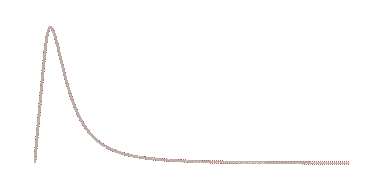α ∈ {1, 2, … }
β ∈ {1, 2, … }
0 ≤ X < ∞
View on StatDist
View on Wikipedia

### `double Gamma(double alpha /* shape */, double beta /* scale */);`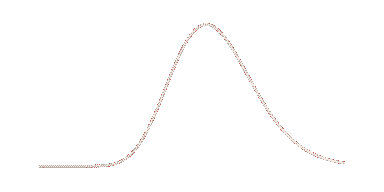0 < α < ∞
0 < β < ∞
0 ≤ X < ∞
View on StatDist
View on Wikipedia

### `double Laplace(double alpha /* location */, double mu /* scale */);`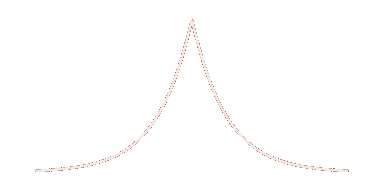0 < α < ∞
-∞ < μ < ∞
-∞ < X < ∞
View on StatDist
View on Wikipedia

### `double Logistic(double mu /* mean */, double sigma /* scale */);`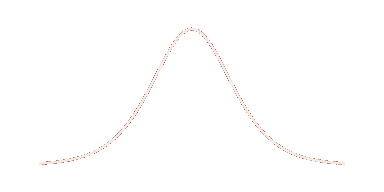-∞ < μ < ∞
0 < σ < ∞
-∞ < X < ∞
View on StatDist
View on Wikipedia

### `double Lognormal(double mu /* mean */, double sigma /* standard deviation */);`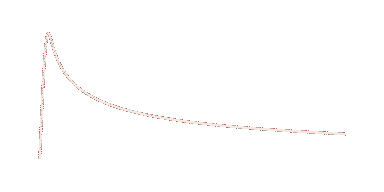-∞ < μ < ∞
0 ≤ σ < ∞
0 ≤ X < ∞
View on StatDist
View on Wikipedia

### `double Normal(double mu /* mean */, double sigma /* standard deviation */);`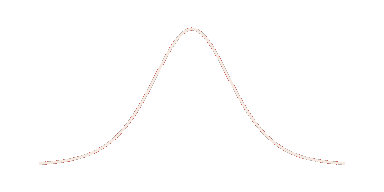-∞ < μ < ∞
0 < σ < ∞
-∞ < X < ∞
View on StatDist
View on Wikipedia

### `double Pareto(double alpha /* scale */, double beta /* shape */);`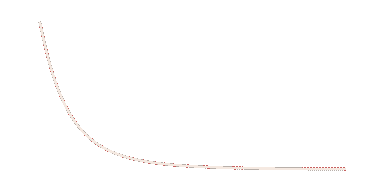0 < α < ∞
0 < β < ∞
α ≤ X < ∞
View on StatDist
View on Wikipedia

### `int Poisson(double lambda /* rate */);`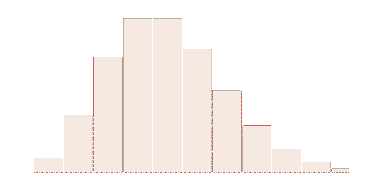0 < λ < ∞
X ∈ { 0, 1, … }
View on StatDist
View on Wikipedia

### `double Rayleigh(double sigma /* scale */);`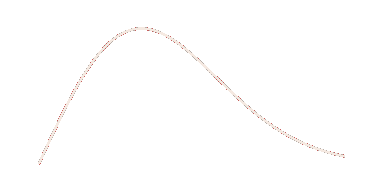0 < σ < ∞
0 ≤ X < ∞
View on StatDist
View on Wikipedia

### `double StudentsT(int nu /* degrees of freedom */);`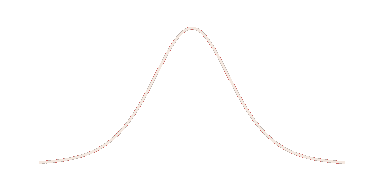ν ∈ { 1, 2, … }
-∞ < X < ∞
View on StatDist
View on Wikipedia

### `double Triangular(double alpha /* min */, double beta /* mode */, double gamma /* max */);`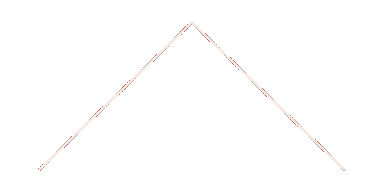-∞ < α < β
α < β < ∞
α ≤ γ ≤ β
α ≤ X ≤ β
View on StatDist
View on Wikipedia

### `double Weibull(double alpha /* shape */, double lambda /* scale */);`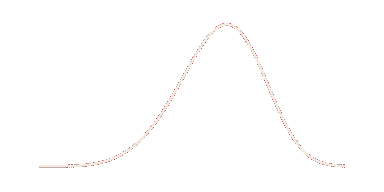0 < α < ∞
0 < λ < ∞
0 ≤ X < ∞
View on StatDist
View on Wikipedia

1. Sorry, gamedev examples only, but the general technique could come in useful for all kinds of C# projects. return ︿

2. Note that when you input an 𝑥, StatDist will plot P(𝒳 < 𝑥), which is more similar to our `luck` stat. If you want to think in terms of a `difficulty` check instead, you’d need to use P(𝒳 > 𝑥). In practice, to switch between them you can simply invert the test result. return ︿

3. You can explore this relationship by looking at the cumulative distribution function graph on the right side of StatDist’s UI. return ︿